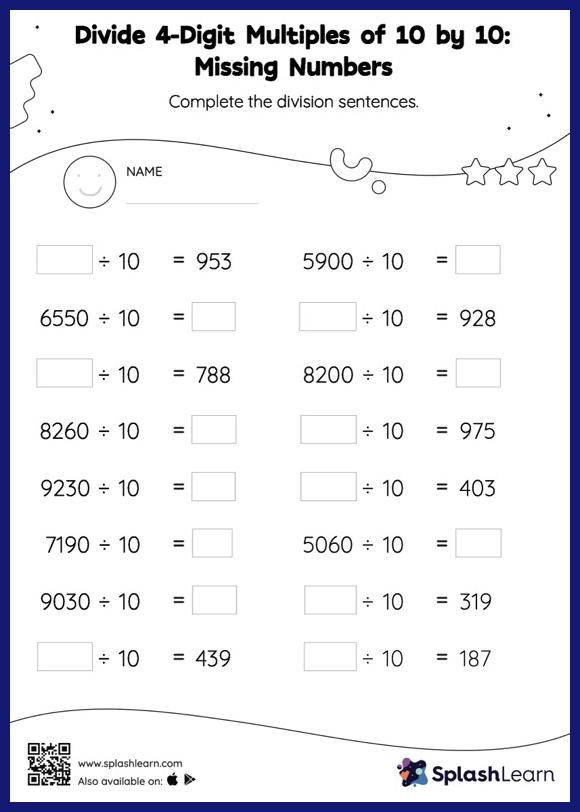# Divide 4-Digit Multiples of 10 by 10: Missing Numbers Worksheet

Home > Divide 4-Digit Multiples of 10 by 10: Missing NumbersStudents look at the number of zeros at the end of the dividend or divisor to find the quotient when dividing by 10 or its multiples. They find the missing number in divide 4-digit multiples of 10 by 10 worksheet using the same understanding. This worksheet is about practicing with the horizontal format in which numbers are written side by side. To develop flexibility with numbers and operations, students need to have enough practice in this format and not just rely on the vertical/column method.